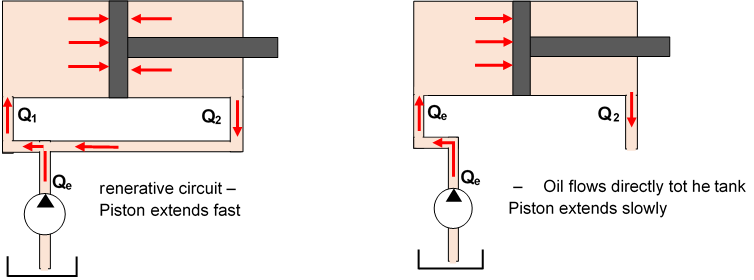# Regenerative circuit### Regenerative Circuit

The so-called regenerative circuit can double the extension speed of a single-rod cylinder without using a larger pump. This means that regeneration circuits save money because a smaller pump, motor, and tank can produce the desired cycle time. It also means that the circuit costs less to operate over the life of the machine.

With this regenerative circuit an empty stroke can be driven at high speed until the workpiece is actually encountered.  When the piston almost reaches the workpiece, it can then be switched to small piston speed. The trick is the 3/2-way valve, which redirects the volume flow from the return line to the inlet side of the cylinder. The following video explains how this hydraulic circuit works:

For simplicity, a schematic representation of this regenerative circuit: This allows us to derive formulas:Fig.: Hydraulic regenerative circuit

A higher output speed can be achieved with a so-called regenerative circuit. Applicable to hydraulic cylinders with piston rod on one side.

Principle: The volume Q2 displaced on the rod side is redirected to the piston side, so that:The oil pressure acts both on the piston side and on the rod side. However, this reduces the resulting piston force. This results:

F1 = p * A1         and    F2 = p * A

=>Fres = F1 – F= p (A1 – A2) = p * Arod

Questions of understanding:

Why can the piston extend within the differential circuit when the same pressure acts on the piston as on the rod side?
Answer: Because the areas on opposite sides of the piston are unequal, the cap end of the cylinder always has more force than the rod side.

Work order:
The hydraulic regenerative control is given with Qe = 20 l/min; p = 80 bar; piston area = 4,00 cm2,  anular area = 2,00 cm2.  Calculate the extending speed with and without redirecting the oil flow as well as the retracting speed! For the load circuit as shown, develop the corresponding electrical control circuit and, alternatively, a PLC control with its program!